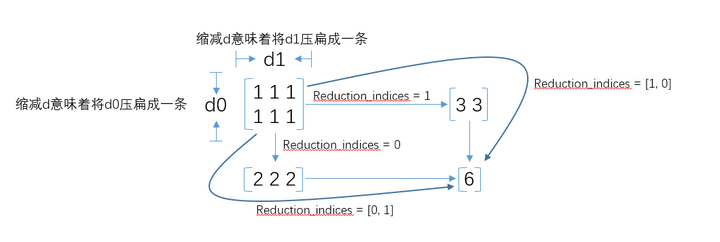## [TensorFlow]Tensor维度理解_tensorflow tensor的维度怎么看_hangkai_tan的博客-程序员秘密

http://wossoneri.github.io/2017/11/15/[Tensorflow]The-dimension-of-Tensor/

### Tensor维度理解

Tensor在Tensorflow中是N维矩阵，所以涉及到Tensor的方法，也都是对矩阵的处理。由于是多维，在Tensorflow中Tensor的流动过程就涉及到升维降维，这篇就通过一些接口的使用，来体会Tensor的维度概念。以下是个人体会，有不准确的请指出。

tf.reduce_mean

``````reduce_mean(
input_tensor,
axis=None,
keep_dims=False,
name=None,
reduction_indices=None
)``````

``````x = tf.constant([[1., 1.], [2., 2.]])
tf.reduce_mean(x)  # 1.5
tf.reduce_mean(x, 0)  # [1.5, 1.5]
tf.reduce_mean(x, 1)  # [1.,  2.]``````

x是二维数组[[1.0,1.0],[2.0, 2.0]]
`axis`参数取默认值时，计算整个数组的均值：(1.+1.+2.+2.)/4=1.5
`axis`取0，意味着对列取均值：[1.5, 1.5]
`axis`取1，意味着对行取均值：[1.0, 2.0]

``````sess = tf.Session()
x = tf.constant([[1., 2., 3.], [4., 5., 6.], [7., 8., 9.]])
print(sess.run(x))
print(sess.run(tf.reduce_mean(x)))
print(sess.run(tf.reduce_mean(x, 0)))
print(sess.run(tf.reduce_mean(x, 1)))``````

``````[[ 1.  2.  3.]
[ 4.  5.  6.]
[ 7.  8.  9.]]
5.0
[ 4.  5.  6.]
[ 2.  5.  8.]``````

``````sess = tf.Session()
x = tf.constant([[[1., 1.], [2., 2.]], [[3., 3.], [4., 4.]]])
print(sess.run(x))
print(sess.run(tf.reduce_mean(x)))
print(sess.run(tf.reduce_mean(x, 0)))
print(sess.run(tf.reduce_mean(x, 1)))
print(sess.run(tf.reduce_mean(x, 2)))``````

``````[[[ 1.  1.]
[ 2.  2.]]

[[ 3.  3.]
[ 4.  4.]]]``````

``````2.5
[[ 2.  2.]
[ 3.  3.]]
[[ 1.5  1.5]
[ 3.5  3.5]]
[[ 1.  2.]
[ 3.  4.]]``````

`axis`参数取默认值时，依然计算整个数组的均值：(float)(1+2+3+4+1+2+3+4)/8=2.5
`axis`取0，计算方式是：

``````[[(1+3)/2, (1+3)/2],
[(2+4)/2, (2+4)/2]]``````

`axis`取1，计算方式是：

``````[[(1+2)/2, (1+2)/2],
[(3+4)/2, (3+4)/2]]``````

`axis`取2，计算方式是：

``````[[(1+1)/2, (2+2)/2],
[(3+3)/2, (4+4)/2]]``````

tf.reduce_xyz(x, axis=k-1)的结果是对最里面一维所有元素进行求和。
tf.reduce_xyz(x, axis=k-2)是对倒数第二层里的向量对应的元素进行求和。
tf.reduce_xyz(x, axis=k-3)把倒数第三层的每个向量对应元素相加。

``````[[[ 1.  1.]
[ 2.  2.]]

[[ 3.  3.]
[ 4.  4.]]]``````

axis=3-1=2，做最内层计算，我们的最内层就是(1,1),(2,2),(3,3),(4,4)，计算出来的就是

``````[[ 1.  2.]
[ 3.  4.]]``````

axis=3-2=1，做倒数第二层计算(参考二维计算)：([1,1],[2,2])和([3, 3],[4, 4])

``````[[ 1.5  1.5]
[ 3.5  3.5]]``````

axis=3-3=1，做倒数第三层计算:([[1, 1], [2, 2]])([[3, 3], [4, 4]])

``````[[ 2.  2.]
[ 3.  3.]]``````

``````# input 4-D
[[[[ 1.  1.]
[ 2.  2.]]

[[ 3.  3.]
[ 4.  4.]]]

[[[ 5.  5.]
[ 6.  6.]]

[[ 7.  7.]
[ 8.  8.]]]]
# axis=none
4.5

# axis=0
[[[ 3.  3.]
[ 4.  4.]]

[[ 5.  5.]
[ 6.  6.]]]

# axis=1
[[[ 2.  2.]
[ 3.  3.]]

[[ 6.  6.]
[ 7.  7.]]]``````

the rank of the tensor is reduced by 1 for each entry in axis``````# 2*2
[[ 1.  1.]
[ 2.  2.]]
# keep_dims=False
[ 1.5  1.5] # 1*2
[ 1.  2.]   #1*2
# keep_dims=True
[[ 1.5  1.5]]   #1*2
[[ 1.]          #2*1
[ 2.]]``````

### 定义数组并赋值_定义一个数组并赋值__Lyang_的博客-程序员秘密

1.定义一个给定长度的字符数组：String[] sz=new String;2.定义一个给定长度的字符数组并对其进行初始化：String[] sz=new String[]{"1","2","3","4","5"};

### 结构体指针初始化问题_zhongyoubing的博客-程序员秘密

（1）结构体指针是否需要初始化struct student{char* name;int score;struct student* next;}stu,*stu1;stu.name=(char*)malloc(sizeof(char));  //1 结构体成员指针需要初始化strcpy(stu.name,"Jim");stu.scor=90;s